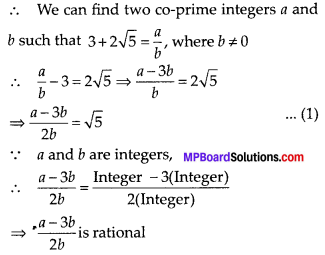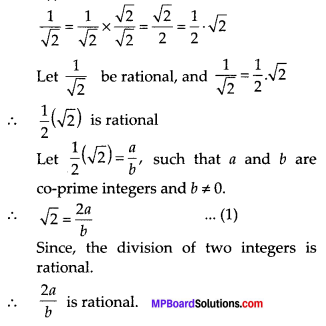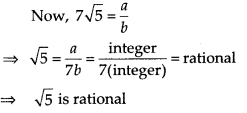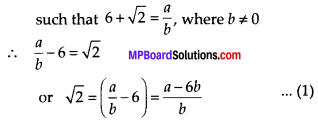# MP Board Class 10th Maths Solutions Chapter 1 Real Numbers Ex 1.3

In this article, we will share MP Board Class 10th Maths Book Solutions Chapter 1 Real Numbers Ex 1.3 Pdf, These solutions are solved subject experts from the latest edition books.

## MP Board Class 10th Maths Solutions Chapter 1 Real Numbers Ex 1.3

Question 1.
Prove that $$\sqrt{5}$$ is irrational.
Solution:
Let us assume, to the contrary, that $$\sqrt{5}$$ is rational.
∴ $$\sqrt{5}=\frac{a}{b}$$
∴ b × $$\sqrt{5}$$ = a
By Squaring on both sides,
5b2 = a2 …………. (i)
∴ 5 divides a2.
5 divides a.
∴ We can write a = 5c.
Substituting the value of ‘a’ in eqn. (i),
5b2 = (5c)2 = 25c2
b2 = 5c2
It means 5 divides b2.
∴ 5 divides b.
∴ ‘a’ and ‘b’ have at least 5 as a common factor.
But this contradicts the fact that a’ and ‘b’ are prime numbers.
∴ $$\sqrt{5}$$ is an irrational number.Question 2.
Prove that 3 +2$$\sqrt{5}$$ is irrational.
Solution:
Let 3 + 2$$\sqrt{5}$$ is rational.⇒ From (1), $$\sqrt{5}$$ is rational
But this contradicts the fact that $$\sqrt{5}$$ is irrational.
∴ Our supposition is wrong.
Hence, 3 + 2$$\sqrt{5}$$ is irrational.Question 3.
Prove that the following are irrationals.
(i) $$\frac{1}{\sqrt{2}}$$
(ii) 7$$\sqrt{5}$$
(iii) 6 + $$\sqrt{2}$$
Solution:
(i) We haveFrom (1), $$\sqrt{2}$$ is rational number which contradicts the fact that $$\sqrt{2}$$ is irrational.
∴ Our assumption is wrong.
Thus, $$\frac{1}{\sqrt{2}}$$ is irrational.

(ii) Let $$7 \sqrt{5}$$ is rational.
∴ We can find two co-prime integers a and b such that $$7 \sqrt{5}=\frac{a}{b}$$, where b ≠ 0This contradicts the fact that $$\sqrt{5}$$ is irrational.
∴ Out assumption is wrong.
Thus, we conclude that 7$$\sqrt{5}$$ is irrational.

(iii) Let 6 + $$\sqrt{2}$$ is rational.
∴ We can find two co-prime integers a and b= Rational [ ∵ a and b are integers]
From (1), $$\sqrt{2}$$ is a rational number,
which contradicts the fact that $$\sqrt{2}$$ is an irrational number.
∴ Our supposition is wrong.
⇒ 6 + $$\sqrt{2}$$ is an irrational number.